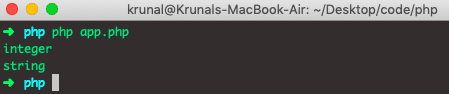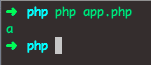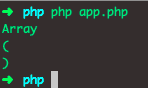# PHP array_keys: How to Get Array Keys in PHP

PHP array_keys() function returns an array containing the keys.

## PHP array_keys()

PHP array_keys() is a built-in function in PHP and is used to return either all the keys of an array or the subset of the keys. If a search value is specified, only the keys for that value are returned. Otherwise, all the keys from an array are returned as the output. In addition, it determines if strict comparison (===) should be used during the search.

### Syntax

The syntax for the array_keys is the following.

`array_keys(array,value,strict)`

### Arguments

An array parameter is required, and it specifies the array.

The value parameter is optional, and you can specify a value, then only the keys with this value are returned.

The strict parameter is optional and used with the value parameter.

### Example

See the following example.

```<?php

// app.php

\$data = ['a' => 'krunal',
'b' => 'ankit',
'd' => 'khushbu',
'f' => 'nehal',
'c' => 'rushabh',
'k' => 'krunal',
'n' => 'nehal'];
\$output = array_keys(\$data);
print_r(\$output);```

So, we get the array of keys.

One thing to note about that if you have keys that are a long integer, such as ‘19214662291595‘, they will be considered as such on the 64bits system but will be of type string on the 32 bits system.

See the following example.

```<?php

// app.php

\$appKeys = array('19214662291595' => null, 'AppDividend291595' => null);

foreach(array_keys(\$appKeys) as \$key){
echo gettype(\$key)."\n";
}
```

See the output.Here’s how to get the first key and last key. See the below code.

Let’s get the first key of an array. For that, we can use the PHP array_shift function.

```<?php

// app.php

\$data = ['a' => 'krunal',
'b' => 'ankit',
'd' => 'khushbu',
'f' => 'nehal',
'c' => 'rushabh',
'k' => 'krunal',
'n' => 'nehal'];
\$keys = array_keys(\$data);
\$firstKey = array_shift(\$keys);
echo \$firstKey."\n";```

See the output.We can also get the last key. For that, we can use the PHP array_pop().

See the below code.

```<?php

// app.php

\$data = ['a' => 'krunal',
'b' => 'ankit',
'd' => 'khushbu',
'f' => 'nehal',
'c' => 'rushabh',
'k' => 'krunal',
'n' => 'nehal'];
\$keys = array_keys(\$data);
\$firstKey = array_pop(\$keys);
echo \$firstKey."\n";```

We will get the in the output because the last key is n.

## Using the strict parameter

Now, let’s use the strict comparison and see the difference in the outputs.

```<?php

// app.php

\$app = array(10,20,30,21);
print_r(array_keys(\$app,"21",true));```

In the above code, we compare the string 21 to the integer 21. The value is the same, but the data type is different.

Also, we are comparing the values under the strict flag. So the output will not be matched, and we see an empty array.If we pass the false parameter, it will give us the key of an array. See the below code.

```<?php

// app.php

\$app = array(10,20,30,21);
print_r(array_keys(\$app,"21",false));```

See the output.One thing to note about that array_keys does not maintain the data type of the keys when mapping them to the new array. This will create an issue with the in_array and lookup on the string characters.

There’s a lot of multidimensional array_keys function out there, but each of them only merges all the keys in one flat array.

That’s it for this tutorial.

Categories PHP

This site uses Akismet to reduce spam. Learn how your comment data is processed.